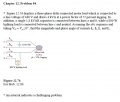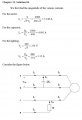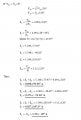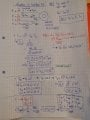# Problem: three phase delta connected balanced motor load, find Ia,Ib,Ic,In

#### Neophyte_2.0

Joined Aug 21, 2019
14
So this is the problem and the solution from the text, but I don't really understand this solution and tried it myself. But while trying myself I found other values (IaM, IbM, IcM) and I didn't know how to calculate Vab#### MrAl

Joined Jun 17, 2014
7,673
Hi,

Do you know where the square root of 3 factor comes from?
That is part of the key to solving this problem.
Hint: the spec's are in line to neutral voltage but the motor runs on line to line voltages.

#### Neophyte_2.0

Joined Aug 21, 2019
14
Hi,

Do you know where the square root of 3 factor comes from?
That is part of the key to solving this problem.
Hint: the spec's are in line to neutral voltage but the motor runs on line to line voltages.
Hi, no I don't really know where the square root of 3 comes from in the conversion of a phase voltage/current to a line voltage/current.
So the motor is connected in delta and I know that with a delta load the phase voltage is the same as the line voltage. So can I say that the phase voltages for the motor (with pf=0,72 (lagging)) are Vab= 440<-43,95° ; Vbc=440<-163,95° ; Vca=440<76,05° ?

#### MrAl

Joined Jun 17, 2014
7,673
Hello again,

Well for now i think as long as you know that the square root of 3 relates the line to neutral voltage to the line to line voltage you're doing just fine. So can you see where one of the calculations comes from now?

The next thing then is to take the line to neutral voltage and convert it to line to line voltage because that's what the motor is running off of.

#### Neophyte_2.0

Joined Aug 21, 2019
14
Hello again,

Well for now i think as long as you know that the square root of 3 relates the line to neutral voltage to the line to line voltage you're doing just fine. So can you see where one of the calculations comes from now?

The next thing then is to take the line to neutral voltage and convert it to line to line voltage because that's what the motor is running off of.
So given is: V(line to line)=440 V and V(an)= V(phase)<0°. But the motor is connected in delta and in delta V(line to line) = V(delta) therefore: Van = 440<0° ?? I don't understand why the factor square root of 3 is necessary here (since the motor is connected in delta) ?

#### ericgibbs

Joined Jan 29, 2010
10,213

#### Neophyte_2.0

Joined Aug 21, 2019
14
But is my method for calculation correct? and if not, what would be the correct method ? Because I've found different methods on the internet with al different solutions for this problem. So I'm very skeptical.

#### Neophyte_2.0

Joined Aug 21, 2019
14
But is my method for calculation correct? and if not, what would be the correct method ? Because I've found different methods on the internet with al different solutions for this problem. So I'm very skeptical.
PS: I don' think the solution from the text is correct, as the solution manual is known to be full of mistakes.

#### MrAl

Joined Jun 17, 2014
7,673
Hello again,

Let's take one at a time.
Do you understand how they got:
IL=S/(sqrt(3)*VL)
?

#### Neophyte_2.0

Joined Aug 21, 2019
14
Hello again,

Let's take one at a time.
Do you understand how they got:
IL=S/(sqrt(3)*VL)
?
yes, that I understand. And is it because there is a neutral line that you can assume that the voltage source is wye(Y) connected? and therefore: Vp= (440/sqrt(3))= 254V ? and Van=Vp<0° = 254<0° ?

Last edited:

#### MrAl

Joined Jun 17, 2014
7,673
yes, that I understand. The first calculation that I don't understand is the one for the lighting: Vp= (440/sqrt(3)), I would say that the voltage across the lighting load is Vcn and since Van=Vp<0° ==> Vbn=Vp<-120° and Vcn=Vp<120°. And because motor is delta connected Vl=Vp=440V, therefore: Van=440<0° , Vbn=440<-120° and Vcn=440<120°, no ?
Hi,

Why on earth would you think that the line to neutral voltage would be the same as the line to line voltage?
Did you look at the phasor drawing for three phase? You can see right away that the distance between the tips of the line to neutral arrows are farther apart than the length of one of the phase arrows. That means the line to line voltage is always greater than the line to neutral voltage. Since the relationship is VLL/sqrt(3)=VLN the line to neutral voltage is equal to the line to line voltage divided by the square root of 3.

Since the lighting load is connected line to neutral, the voltage across that must be less than the line to line voltage of 440vac.

If this does not make sense, then look at the phasor diagram and note also that the line to line resultants form a perfect equilateral triangle the sides of which will always be longer than the distance from the center to any vertex, and are related by the square root of three.

#### Neophyte_2.0

Joined Aug 21, 2019
14
Hi,

Why on earth would you think that the line to neutral voltage would be the same as the line to line voltage?
Did you look at the phasor drawing for three phase? You can see right away that the distance between the tips of the line to neutral arrows are farther apart than the length of one of the phase arrows. That means the line to line voltage is always greater than the line to neutral voltage. Since the relationship is VLL/sqrt(3)=VLN the line to neutral voltage is equal to the line to line voltage divided by the square root of 3.

Since the lighting load is connected line to neutral, the voltage across that must be less than the line to line voltage of 440vac.

If this does not make sense, then look at the phasor diagram and note also that the line to line resultants form a perfect equilateral triangle the sides of which will always be longer than the distance from the center to any vertex, and are related by the square root of three.
Because I looked at it from the perspective of the motor load which is delta connected. So I thought for delta: Vl=Vp and given is that Van=Vp<0° so therefore Van=Vl<0°. But I realise now that I can assume that the source is wye(Y) connected (because of the presence of a neutral line) and work from there

#### MrAl

Joined Jun 17, 2014
7,673
Because I looked at it from the perspective of the motor load which is delta connected. So I thought for delta: Vl=Vp and given is that Van=Vp<0° so therefore Van=Vl<0°. But I realise now that I can assume that the source is wye(Y) connected (because of the presence of a neutral line) and work from there
Oh yes the source is wye. That means the voltage line to neutral is 440/sqrt(3).
BTW the lower case "L" shows up looking almost like an upper case "I" or a numeral "1" so maybe you should use upper case "L" or just use Courier New font.

Also, in my experience we always used VLL for voltage line to line not VL (line voltage) because sometimes "line voltage" means the line to neutral voltage. "Voltage line to line" is harder to confuse with "Voltage line to neutral" so one would be VLL and the other VLN or written with a subscript V_LL and V_LN.

#### MrAl

Joined Jun 17, 2014
7,673
Another good exercise and a method to check your work is as follows.

Figure out what each phase of the motor looks like in terms of a resistor in parallel (or in series) with an inductor. Since the motor is a balanced load the three phases will all have the same values of R and L.
Solve for the resistor value first, which will be the real power absorber, then solve for the value of the inductor at whatever frequency you want to use (w=1 is a good choice for this). Also convert the capacitor to have a real capacitor value like 9uf or something.
Once you have this R and L, you can construct the entire circuit and check your currents using some basic concepts of circuit analysis. You will also have to transform the three wye sources into their mathematical forms.

This i believe is a really good exercise if you have the time. This will teach you the basic concepts about how to analyze any three phase system even if it is unbalanced. You can also then use your R and L and some sine sources to check everything in a circuit simulator also which is of great benefit when you want to be sure you have the right results.

•Neophyte_2.0

#### MrAl

Joined Jun 17, 2014
7,673
I thought i would update a little because i did some calculations and simulations.
What i found is that it looks like the text is wrong when it comes to Ia, Ib, and Ic.
What we need now is a 3rd party to repeat the simulations and see if they find the same.
Anybody interested?

[MUCH LATER]
I redid the calculations and simulations and the results of the two agree to close approximation.
Since the results of both of these does not match the values in the text for Ia, Ib, and Ic, i can only believe that the values shown in the text are not correct. In fact, they are very much different.
I guess the next step would be to try to figure out how they could have come up with those results they must have done something wrong like some bad assumption.

Last edited: